Electrochemistry 1

For on-line tutorial help with this topic, refer to the chemcal module "Electrochemistry".

Shortcut to Questions

Q: 1 2 3 4 5 6 7 8 9 10 11

1

(a) Define the term standard electrode potential.

(b) How can it be shown that the standard electrode potential for the system

Ga3+ + 3e-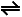Ga

is -0.53 volt?

(c) If the standard electrode potential for the system

Ag+ + e-Ag

is + 0.80 volt, determine the standard emf of a cell with the electrodes in (b) and (c) and give equations for the reactions that take place at each electrode.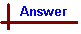2

Write the equation for the two half cell reactions, the net cell reaction and calculate the cell voltage for each of the following cells:

(a) Pt l H2(1 atm) l H+(1 M) ll Cl-(1 atm) l Cl2(g) l Pt

(b) Zn l Zn2+(1 M) ll H+(1 M) l H2(1 atm) l Pt3

An electrochemical cell is set up as in the diagram -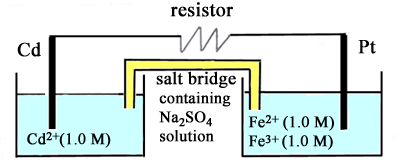(a) Write the equation for the reaction at the oxidation electrode (anode)

(b) Write the equation for the reaction at the reduction electrode (cathode).

(c) What is the potential difference between the two electrodes before the resistor is connected between them?

(d) What is the potential difference between the two electrodes when chemical equilibrium has been established?

(e) What will be the change in mass of the cadmium rod after 0.0100 mole of electrons have passed through the circuit? Indicate whether this change is an increase or a decrease.4

(a) Draw a diagram for an electrochemical cell composed of a standard hydrogen half cell and a half cell in which a silver rod is in contact with 1.00 M silver nitrate solution. Indicate the oxidation electrode, the reduction electrode, the direction of electron flow and the direction of ion migration when the electrodes are connected by a wire.

(b) Write the equation for the spontaneous reaction at the oxidation electrode (anode).

(c) Write the equation for the spontaneous reaction at the reduction electrode (cathode).

(d) What is the potential difference between the two electrodes?

(e) What change occurs in the potential difference when solid sodium chloride is added to the silver nitrate solution?

Write "no change", "increase" or "decrease".5

Any one of the halogen molecules or ions might react with iron(II) or iron(III) ions in solution. Using the appropriate standard electrode potentials, write a balanced equation for any reactions that you predict would occur.

Standard electrode potentials Eo V
F2 + 2e-2F- +2.87 V
Cl2 + 2e-2Cl- +1.40 V
Br2 + 2e-2Br- +1.09 V
Fe3+ + e-Fe2+ +0.77 V
I2 + 2e-2I- +0.62 V6

Silver(I) ion is a weak oxidant. Using the code letters provided, arrange the following half cells in order of increasing electrode potential -

1 Ag l AgCH3CO2 (0.0010 M)

2 Ag l AgCl (saturated)

3 Ag l AgCl (saturated, and then made 2.0 M with respect to NH3)

4 Ag l AgCl (saturated, and then made 0.10 M with respect to NH3)

5 Ag l AgNO3 (1.0 M)7
 Standard electrode potentials Eo V Au+ + e-Au +1.7 Au3+ + 3e-Au +1.5 Au3+ + 2e-Au+ +1.3 Fe3+ + e-Fe2+ +0.8

Using the above abbreviated table, write an equation for the reaction which occurs in each of the following cases. If there is no reaction, write "no reaction".

(a) 1 M Au3+ solution and 1 M Fe2+ solution are mixed.

(b) 1 M Au3+ solution and 1 M Fe3+ solution are mixed.

(c) Au metal is added to 1 M Au3+ solution.

(d) Comment on the possibility of preparing a stable 1 M water solution of a gold(I) salt. Give reasons for your answer.8

The diagram below represents an electrochemical cell.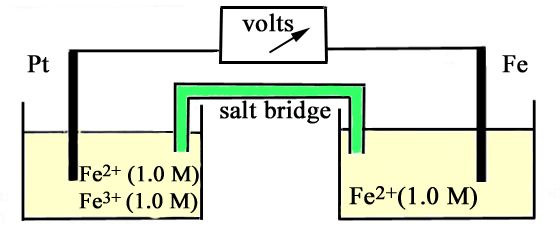(a) Write the half-cell equation for the reduction electrode reaction.

(b) Write the half-cell equation for the oxidation electrode reaction.

(c) Write the equation for the net cell reaction.

(d) What is the value of the e.m.f. between the Pt and Fe rods?9

The following diagram represents an electrochemical cell, composed of two half cells, linked through a potassium chloride salt bridge and a voltmeter.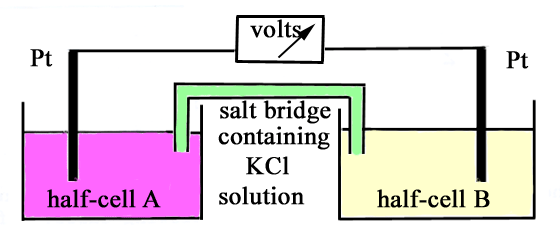(a) In the table below are given the constituents of each half cell. In each case predict the net reaction, if any, which will occur. Write the equation for the net reaction, and give the value of the standard potential of the cell, Eo(cell). If you predict no reaction occurs, write "no reaction".

 Half-cell A Half-cell B (i) Fe3+(1 M),Fe2+(1 M) l Pt Sn4+(1 M),Sn2+(1 M) l Pt (ii) MnO4-(1 M),H+(1 M),Mn2+(1 M) l Pt Fe3+(1 M),Fe2+(1 M) l Pt (iii) UO22+(1 M),UO2+(1 M) l Pt V3+(1 M),V2+(1 M) l Pt

(b) Explain the purpose of the salt bridge.10

An electrochemical cell is set up using the couples Pt l Fe3+,Fe2+ and Zn2+ l Zn. All species are present in their standard states.

(a) Draw a diagram to illustrate such a cell, and indicate on it all relevant information to show how it works.

(b) What is the potential difference of this cell?

(c) After the cell has operated for a time, the zinc electrode is found to have changed in mass by 0.327 gram. Find the change in the number of mole of

(i) Fe2+, (ii) Fe3+ and indicate whether the amount is an "increase" or a "decrease".11

Consider an electrochemical cell made by connecting the following two half cells -

(a) a cobalt rod immersed in 1 M cobalt(II) nitrate,

(b) a carbon rod immersed in 1 M hydrochloric acid and with chlorine gas at 1 atmosphere pressure being bubbled around the rod.

When the electrodes are connected by a voltmeter it is observed that the cell potential is 1.64 volts, and that the cobalt electrode is negative.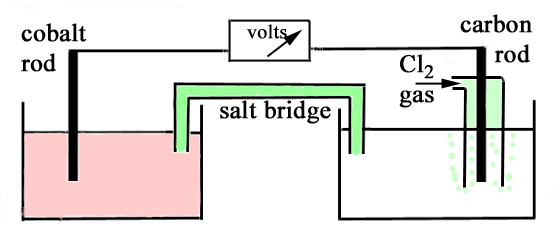(a) Write the oxidation half equation for the cell reaction

(b) Write the reduction half equation for the cell reaction

(c) Write the equation for the cell reaction

(d) What is the standard electrode (reduction) potential for the cobalt(II)/cobalt couple?

(e) Indicate clearly on the diagram of the functioning cell:

(i) the direction of electron flow

(ii) the directions of +ve ion and -ve ion migration in the bridge

(iii) the oxidation electrode (anode)

(iv) the reduction electrode (cathode)

(f) How would the cell voltage change if the concentration of cobalt(II) nitrate in solution were decreased? (Write "increased", "decreased", or "no change").1

(a) The standard electrode potential (Eo) of a half-cell system (electrode) represented as

Mn+ + ne-M is the potential of the right hand electrode less that of the left hand electrode in the cell

Pt l H2(g)(1 at) l H+(1 M) ll Mn+(1 M) l M

All the components are in their standard states - i.e. solutes have a concentration of 1 M and gases are at a pressure of 1 atmosphere pressure (strictly, 100 kPa).

(b) Connect the standard Ga3+ l Ga electrode with a standard hydrogen electrode and observe the potential difference between the two half cells. The Ga electrode will be found to be negative with respect to the standard hydrogen electrode and the potential difference between the two electrodes will be 0.53 volt.

(c) The potential difference for any cell whose components are all in their standard states can be calculated from the tables of standard electrode potentials (also known as standard reduction potentials) as follows:

Eocell = Eo(larger) - Eo(smaller) = Eo(reduction) - Eo(oxidation).

Eo = (+0.80) - (-0.53) V = +1.33 V

N.B. Be careful with the signs of the component Eo values in the equation. Note that the sign for the cell potential difference calculated must be positive as this means that the reaction is spontaneous.

To determine the reaction direction, the half-cell with the larger Eo value is the one which undergoes reduction while oxidation occurs in the half-cell with the smaller Eo.

Thus as the Ag+ l Ag half-cell has the larger Eo value, reduction occurs in the Ag+ l Ag half-cell:

Ag+ + e- → Ag

while oxidation occurs in the Ga3+ l Ga half-cell:

Ga → Ga3+ + 3e-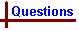2

(a) The Eo for the standard Cl2(g) l Cl- half-cell = 1.36 V while Eo for the standard hydrogen half-cell to which it is connected is zero by definition.

Therefore reduction occurs in the Cl2(g) l Cl- half-cell and oxidation occurs in the hydrogen half-cell according to the following equations:

Cl2(g) + 2e- → 2Cl-

H2(g) → 2H+ + 2e-

and the overall cell reaction is

Cl2(g) + H2(g) → 2Cl- + 2H+

The cell voltage = Eocell = Eo(red) - Eo(ox).

Eo = (+1.36) - (-0.00) V = +1.36 V

(b) The Eo for the standard Zn2+ l Zn half-cell = -0.76 V while Eo for the standard hydrogen half-cell to which it is connected is zero by definition.

Therefore reduction occurs in the hydrogen half-cell and oxidation occurs in the Zn2+ l Zn half-cell according to the following equations:

2H+ + 2e- → H2(g)

Zn → Zn2+ + 2e-

and the overall cell reaction is

Zn + 2H+ → Zn2+ + H2(g)

The cell voltage = Eocell = Eo(red) - Eo(ox).

Eo = (+0.00) - (-0.76) V = +0.76 V3

The two cell half-reactions and their Eo values are

Cd2+ + 2e-Cd Eo = -0.40 V

Fe3+ + e-Fe2+ Eo = + 0.77 V

As the Eo for the Fe3+,Fe2+ half-cell is larger, this will be the reduction (cathode) and the oxidation will occur in the Cd2+ l Cd half-cell (anode).

(a) Anode reaction: Cd → Cd2+ + 2e-

(b) Cathode reaction: Fe3+ + e- → Fe2+

(c) Eo(cell) = Eo(red) - Eo(ox)

= (+0.77) - (-0.40) V

= 1.17 V

(d) After the resistor is connected, electrons can flow from the anode to the cathode via the resistor and the reactions can proceed in both cells. Equilibrium will occur when all of the reactants in each cell have been consumed and then the voltage will be zero.

(e) From the equation above, one mole of electrons passing through the cells causes ½ mole of Cd to be oxidized to Cd2+.

∴ 0.0100 mole of electrons would cause 0.00500 mole of Cd to be oxidized.

Mass of cadmium oxidized = 0.00500 x 112.4 g = 0.562 g (decrease in mass).4

(a) The half-cell reactions and their standard Eo values are:

2H+ + 2e-H2       0.00 V

Ag+ + e-Ag       +0.80 V

As the Eo for the Ag+ l Ag half cell is larger, this half cell will undergo reduction so it is the cathode in the cell while the H+ l H2 half cell is the anode and it undergoes oxidation.

Electrons will flow through the wire from the half-cell where oxidation occurs to the half-cell where reduction occurs, i.e. from the hydrogen half-cell to the silver half-cell.

In the hydrogen half-cell, H2 molecules are oxidized to H+ ions, so there will be excess positive ions in that half cell while in the silver half-cell, Ag+ ions are reduced to Ag atoms, creating a deficiency of positive ions there. To maintain electrical neutrality, negative ions move from the bridge to the hydrogen half-cell and positive ions move from the bridge to the silver half-cell.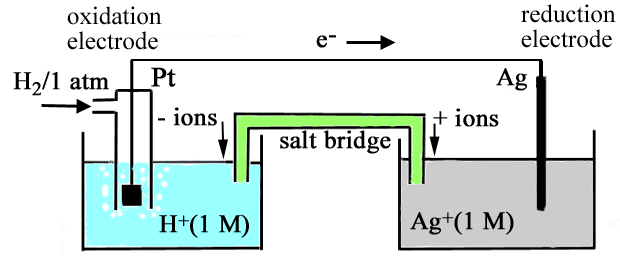(b) Anode reaction: H2 → 2H+ + 2e- (oxidation)

(c) Cathode reaction: Ag+ + e- → Ag (reduction)

(d) Eo(cell) = Eo(red) - Eo(ox)

= (+0.80) - (0.00) = +0.80 V

(e) Addition of Cl- to the silver half-cell causes silver chloride to precipitate and thus reduces the concentration of Ag+ in the silver half-cell. Lowering the [Ag+] causes the equilibrium in the cell, represented by the equation

2Ag+ + H22Ag + 2H+

to move to the left.

i.e. there is less tendency for the reaction to occur and the cell voltage will be smaller.5

As Eo for the F2 l F-, Cl2 l Cl- and Br2 l Br- systems > Eo for Fe3+,Fe2+ system, then these three halogen systems will undergo reduction and cause the Fe3+,Fe2+ system to be oxidized.

However, Eo for the I2 l I- system is < Eo for the Fe3+,Fe2+ system so for this combination, the Fe3+,Fe2+ system will undergo reduction while the I2 l I- system will undergo oxidation.

[Note that if the Eo tables are written with the largest values at the top, a reduction (L → R) can be brought about by an oxidation (R → L) below it in the table, but not by an oxidation above it.]

The reaction equations are as follows:

2Fe2+ + F2 → 2Fe3+ + 2F-

2Fe2+ + Cl2 → 2Fe3+ + 2Cl-

2Fe2+ + Br2 → 2Fe3+ + 2Br-

2Fe3+ + 2I- → 2Fe2+ + I26

In each system, the half-cell reaction is

Ag+ + e-Ag

For standard conditions where [Ag+] is 1.00 M, Eo = +0.80 V

System 5 has this concentration. Each of the other half-cell systems has a smaller [Ag+].

Lowering the [Ag+] causes the equilibrium in the half-cell to move to the left.

i.e. there is less tendency for the reaction to occur and the half-cell voltage will be smaller than in half-cell 5. Thus the ranking for the half-cell E values is just the ranking of the concentrations of Ag+ in each half-cell.

The solubility product constants for AgCl (half-cell 2) and AgCH3CO2 (half-cell 1) have the same units as both salts have the same general formula, AgX and therefore their values can be compared directly. The values are 2 x 10-10 M2 and 3 x 10-3 M2 respectively, so [Ag+] is greater in the silver acetate solution. Hence E for system 1 < E for system 2.

In systems 3 and 4 the concentration of Ag+ is reduced even further than that in system 2 because ammonia combines with silver ion to form the complex, diamminesilver(I) ion and reduces the free silver ion concentration to very low levels.

Ag+ + 2NH3[Ag(NH3)2]+

The larger the [NH3] in the solution, the smaller will be the [Ag+].

Thus the overall ranking of [Ag+] and therefore the E values for the half-cell systems is:

3 < 4 < 2 < 1 < 57

(a) A redox reaction between Au3+ and Fe2+ can occur as Eo for the Fe3+,Fe2+ system is smaller than Eo for either of the two possible reductions of Au3+ listed. Of the two possible reductions of Au3+, the reduction to form Au rather than Au+ will occur as it has the larger Eo value.

Au3+ + 3Fe2+Au + 3Fe3+

(b) No reaction can occur as both Au3+ and Fe3+ are fully oxidized.

For a redox reaction to occur, it requires a reduction half-reaction (left to right in the table) to be combined with an oxidation half-reaction (right to left in the table).

(c) No reaction occurs. The potential redox reaction is the result of the combination of the half-reactions

Au3+ + 3e- → Au (a reduction) and

Au → Au+ + e- (an oxidation)

However, Eo for the overall cell reaction would be

Eo = Eo (reduction) - Eo (oxidation) = (+1.5) - (+1.7)

= - 0.2 V.

A negative Eo(cell) indicates that this reaction is not spontaneous and in fact the reverse reaction is the spontaneous direction.

[When the Eo tables are written with the largest values at the top, a reduction (L → R) can be brought about by an oxidation (R → L) below it in the table, but not by an oxidation above it.]

(d) From the data supplied it can be seen that Au+ can be both reduced (to Au) and oxidized (to Au3+). The half-reactions are

Au+ + e- → Au (reduction of Au+)

Au+ → Au3+ + 2e- (oxidation of Au+)

and the overall reaction is

3Au+ → 2Au + Au3+

The Eo(cell) for the reaction = Eo(reduction) - Eo(oxidation)

= (+1.7) - (+1.3) = 0.4 V

Hence a 1 M solution of an Au+ salt would decompose to Au metal and an Au3+ salt.8

The two cell half-reactions and their Eo values are

Fe3+ + e-Fe2+        Eo = + 0.77 V

Fe2+ + 2e-Fe        Eo = - 0.41 V

(a) As Eo for the Fe3+,Fe2+ system is larger, this half cell will undergo reduction.

Fe3+ + e- → Fe2+

(b) As Eo for the Fe2+ l Fe system is smaller, this half cell will undergo oxidation.

Fe → Fe2+ + 2e-

(c) 2Fe3+ + Fe → 3Fe2+

(d) Eo(cell) = Eo(red) - Eo(ox)

= (+0.77) - (-0.41)

= 1.18 V9

(a) (i) The two cell half-reactions and their Eo values are

Half-cell A: Fe3+ + e-Fe2+ Eo = + 0.77 V

Half-cell B: Sn4+ + 2e-Sn2+ Eo = +0.15 V

As Eo for half-cell A is larger, this half-cell will undergo reduction and half-cell B will undergo oxidation.

Reduction equation: Fe3+ + e- → Fe2+

Oxidation equation: Sn2+ → Sn4+ + 2e-

Net equation: 2Fe3+ + Sn2+ → Sn4+ + 2Fe2+

Cell voltage Eo(cell) = Eo(red) - Eo(ox)

= (+0.77) - (+0.15)

= 0.62 V

(ii) The two cell half-reactions and their Eo values are

Half-cell A: MnO4- + 8H+ + 5e-Mn2+ + 4H2O Eo = + 1.51 V

Half-cell B: Fe3+ + e-Fe2+ Eo = + 0.77 V

As Eo for half-cell A is larger, this half-cell will undergo reduction and half-cell B will undergo oxidation.

Reduction equation: MnO4- + 8H+ + 5e- → Mn2+ + 4H2O

Oxidation equation: Fe2+ → Fe3+ + e-

Net equation: MnO4- + 8H+ + 5Fe2+ → 5Fe3+ + Mn2+ + 4H2O

Cell voltage Eo(cell) = Eo(red) - Eo(ox)

= (+1.51) - (+0.77)

= 0.74 V

(iii) The two cell half-reactions and their Eo values are

Half-cell A: UO22+ + e-UO2+ Eo = + 0.05 V

Half-cell B: V3+ + e-V2+ Eo = - 0.26 V

As Eo for half-cell A is larger, this half-cell will undergo reduction and half-cell B will undergo oxidation.

Reduction equation: UO22+ + e- → UO2+

Oxidation equation: V2+ → V3+ + e-

Net equation: UO22+ + V2+ → V3+ + UO2+

Cell voltage Eo(cell) = Eo(red) - Eo(ox)

= (+0.05) - (-0.26)

= 0.31 V

(b) To retain the ionic charge balance in both cells. This is necessary due to the loss of positively charged ions in half-cell A and the increase of positively charged ions in half-cell B in each case. Ionic charge balance is maintained by K+ ions leaving the salt bridge into half-cell A and Cl- ions leaving the salt bridge into half-cell B. Note that ions do not migrate from half-cell A to half-cell B - if this were to occur, the redox reaction could take place directly as the half-cells would no longer be isolated and no electrons would flow through the wire.10

(a) The two half-cell reactions and their Eo values are

Fe3+ + e-Fe2+       Eo = + 0.77 V

Zn2+ + 2e-Zn       Eo = - 0.76 V

As Eo for the Pt l Fe3+,Fe2+ electrode is larger, this half-cell will undergo reduction.

Fe3+ + e- → Fe2+

As Eo for the Zn2+ l Zn electrode is smaller, this half-cell will undergo oxidation.

Zn → Zn2+ + 2e-

Electrons will flow from the oxidation electrode (anode) to the reduction electrode (cathode).

Positive ions will migrate from the bridge to the Fe3+,Fe2+ half-cell and negative ions will migrate from the bridge to the Zn2+ l Zn half-cell.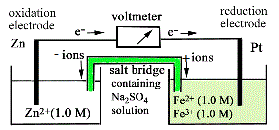(b) Cell voltage Eo(cell) = Eo(red) - Eo(ox)

= (+0.77) - (-0.76)

= 1.53 V

(c) Moles of Zn oxidized = 0.327/65.38 = 5.00 x 10-3 mol.

∴ moles of electrons flowing = 2 x moles of Zn oxidized = 1.00 x 10-2 mol.

One mole of Fe3+ is reduced by one mole of electrons flowing to produce one mole of Fe2+.

∴ moles of Fe3+ reduced = 1.00 x 10-2 mol (decrease)

and moles of Fe2+ formed = 1.00 x 10-2 mol (increase).11

(a) The two half-cell reactions are

Co2+ + 2e-Co

Cl2 + 2e-2Cl-

Cell voltage Eo(cell) = Eo(red) - Eo(ox) = 1.64 V

As the Co2+ l Co half-cell is the negative electrode, this half-cell must be where oxidation occurs (anode) and the

Cl2(g) l Cl- l C half-cell must be the reduction electrode (cathode).

∴ 1.64 = Eo(red) - (-0.28)

Eo(red) = 1.36 V

(b) Co → Co2+ + 2e-

(c) Cl2 + 2e- → 2Cl-

(d) Cl2 + Co → Co2+ + 2Cl-

(e)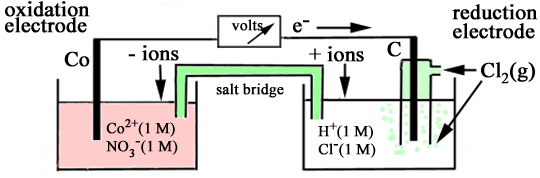(f) The equilibrium reaction for the cell can be represented by the equation

Cl2 + CoCo2+ + 2Cl-

If the [Co2+] were decreased, the equilibrium would shift to the right and thus there would be a greater tendency for the reaction to occur, indicated by an increase in the cell voltage.